# Transistor low frequency amplifier

 Transistor low-frequency amplifiers are mainly used to amplify low-frequency small-signal voltages, with frequencies ranging from tens of hertz to one hundred kilohertz
 First, the bias circuit of the transistor
 In order for the amplifier to achieve linear amplification, the transistor must not only have a suitable static operating point, but must also stabilize the operating point. Due to the influence of temperature on the tube parameters Î², Icbo, Ube, they are finally reflected in the change of Ic. In order to eliminate this effect, we stabilize the static operating point through the negative feedback of the DC or voltage biased by the transistor. The two kinds of bias circuits and working point stability principle are as follows
Table I, Bias circuit of transistor amplifier
Circuit
Working point stability principle
Calculation formula
Negative current feedback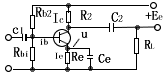Set temperature T â†‘, DC negative feedback process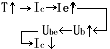As a result, Ic remains unchanged
U = (1 / 3-1 / 5) Ec
Re = (1 / 3-1 / 5) Ec / Ic
Rb = Rb1 // Rb2
=== (2-5) Re
Ub = Rb1Ec / (Rb1 + Rb2)
Voltage negative feedback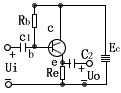Set temperature T, DC negative feedback process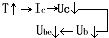As a result, Ic remains unchanged
Rb = Î² (Ec-Ube) / Ic-Î²Rc
Ic = Ec / (Rc + Rb / Î²)
According to experience, usually take
Rb / Rc = (2-10)
 Second, the three circuit forms of the amplifier The amplifier is a three-terminal circuit, one of which must be the common â€œgroundâ€ terminal of the input and output. It is a common circuit, which is connected to the base and is called a common circuit. These three have different performances, see the table below
Three circuit forms and their performance comparison
 Circuit Voltage magnification Current magnification Input resistance Output resistance Common firing circuit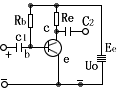10-100 Big 10-1000 Big 100Î©-50KÎ© in 10KÎ©-500KÎ© in Common circuit0.9-0.999 small 10-1000 Big Due to different loads, up to about 50MÎ© 1-100Î© small Common base circuit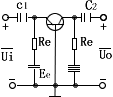100-10000 (practical) Big 0.9-0.999 small About 10-500Î© small 500KÎ©-5MÎ© Big
 3. Graphic method
 The so-called graphical method is to use the input and output characteristic curves of the transistor to analyze the performance of the amplifier by drawing. The graphical method can intuitively and comprehensively indicate the working process of the transistor amplification, and can calculate some performance indicators of the amplifier. Examples to illustrate the graphical process of graphical methods, Example: Knowing the parameters and input voltage Ui = 15sinÏ‰t (millivolt) in the circuit shown in the figure below requires graphically determining the static operating point parameters Ibq, Icq, and Iceq of the circuit, and calculating the voltage and current amplification factors Ku and Kio.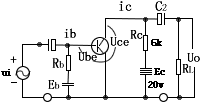Graphical steps 1. Determine the static working point of the base loop, and select the midpoint Q of the straight line segment from the input characteristic curve (Ubeq = 0.7 V at this point, Ibq = 40 Î¼A) as the static working point of the base loop, by selecting the appropriate Eb or Rb (usually by adjusting Rb) to meet the requirements of the working point, 2. Make the DC load line. From the above figure, the load line equation is Uce = Ec-IcRc. Its trajectory is a straight line. If Ic = 0, Uce = Ec = 20 volts, and mark N on the horizontal axis. Point; and let Uce = 0, Ic = Ec / Rc = 20V / 6kohm = 3.3mA, mark M point on the vertical axis, connecting M and N is the DC load line. It intersects with the output characteristic curve of Ib = 40 Î¼A at Q, and finds Icq = 1.8 mA, Uceq = 9 V from point Q, point Q is the static operating point of the collector circuit. For simplicity, the static current , The voltage is no longer added with subscript Q, Ic, Ie is Icq, Ieqo 3. Make a waveform, make a waveform Ut = 15sinÏ‰t (millivolt) on the input characteristics, and make the waveforms of ib, ic, and Uce according to the waveform of Ut. The following points can be obtained from the graphical method (1) The sinusoidality of the waveform can be judged Whether the selection of static operating point Q is appropriate. (2) From the diagram, it is known that the input voltage Ui is opposite to the collector output voltage Uo, and the base current ib and the collector current Ic are in phase with the input voltage Ui. (3) The above graphic method is carried out under no-load conditions. If the effect of the load resistance RL is considered, the AC load should be RL = RC // RL. Since the AC load line and the DC load line both intersect at Q, a straight line M'N 'with an inclination angle a' = (arctg) 1 / RL is made through the Q point, which is called an AC load line. 4. Equivalent circuit method and h parameter 1. The simplified "parameter change" of the equivalent circuit of the h parameter means that the Ib, Ube, Ic, and Uce of the transistor change only slightly in the vicinity of the static operating point Q. Ib and Ube are the input variables of the transistor, and Ic and Uce are the output variables. If you regard the transistor as a two-port network with a controlled source, you can use four h parameters to simulate the physical structure of the transistor, so that the equivalent circuit of the h parameter of the transistor is shown in Figure 7-1-4. The definition of h is as follows : hie = â–³ Ube / â–³ Ib ------- â–³ Uce = 0, --hfe = â–³ Ic / â–³ Ib ----- â–³ Uce = 0 hre = â–³ Ube / â–³ Uce ------ â–³ Ib = 0, --hoe = â–³ Ic / â–³ Uce ---- â–³ Ib = O Several parameters have their own physical meanings: hie is the input resistance when the output is short-circuited, that is, the reciprocal of the slope of the input characteristic curve; hfe is the current amplification factor of the short-circuit at the output, ie Î² (common emitter) or a (common base Pole); hre is the internal feedback coefficient of the open circuit at the input terminal, which indicates the degree of the influence of the output voltage on the input voltage; hoe is the output conductance when the input terminal is open circuit, that is, the slope of the output characteristic curve. When the transistor works at low frequency, hre and The two parameters of hoe are so small that they are negligible. Usually, two parameters of hie and hre are used to simulate the low-frequency transistor circuit. This is called a simplified equivalent circuit of h parameters, as shown in Figure 7-1-3. , Î’ is the above hie, hfe. The current amplification factor Î² (or hfe) can be obtained from the output characteristic curve or tested by the instrument, and the input resistance rbe is calculated by the following formula: rbe = rb + (Î² + 1) 26 (millivolt) / Ie (milliampere) In the formula: Rb is the base resistance, about a few hundred ohms, Ie is the static emitter current. The method to find the micro-variable equivalent circuit of the transistor amplifier is as follows: (1) The equivalent of the transistor shown in Figure 7-1-3 Analog type replacement; (2) All DC power supplies, DC blocking capacitors and bypass capacitors are regarded as short circuits; (3) Other components are drawn according to their original relative positions, The equivalent circuit can be used to find the amplifier's amplification factor, input resistance, output resistance, and analyze the frequency characteristics of the amplifier.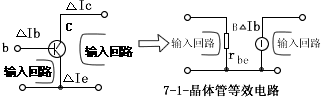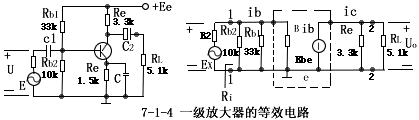The world premiere of the technical zone! ROHM has developed the Power Supply IC "BD372xx series" for high-quality audio. A practical guide for the purchase of home wireless routers. Understanding the circuit diagram and working principle of the audio. Talking about the "frequency response curve" in the audio. Deep dismantling report of the M0pro speaker: both internal and externalInteresting and informative information and technical dry goodsCreate your own personal electronic circleLock the latest course activities and technical live broadcast
Collect People collection
comment
Publish

related suggestion

Posted at 2006-04-16 18:54 â€¢ 241 times read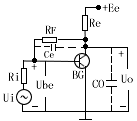var check_allow = "/d/Api/iscantalk.html"; var add_url = '/ d / article / write /'; function CheckLogin () {now_uid = ''; var ElecfansApi_checklogin = '/ webapi / passport / checklogin'; var logout_url = "{: U ('Login / logout')}"; var logout_url = 'http://bbs.elecfans.com/member.php?mod=logging&action=logout&refer=front'; \$ .get (ElecfansApi_checklogin, function (data, textStatus) {if (data! = "") {EchoLoginInfo (data); CheckEmailInfo (data); data = \$ .parseJSON (data); now_uid = data.uid; / * var login_content = 'write an article'+ data.username +'
Quit '; * / var login_content =' write an article'+ data.username +'
Set exit '; \$ (' # login_area '). Html (login_content); var win_width = \$ (window) .width (); if (win_width> 1000) {\$ ("# mine"). MouseDelay (200) .hover (function () {\$ ("# mymenu"). show ();}, function () {\$ ("# mymenu"). hide ();});}} else {var content = 'Login Registration'; \$ ('# login_area'). html (content); \$ (". special-login"). click (function (e) {\$ .tActivityLogin (); return false;});}});} \$ (function () {// comment ------------------------------- var comment = \$ ("# comment"); var comment_input = \$ ("# comContent"); // Submit comment click event interaction \$ ("# comSubmit2"). on ('click', function () {var content = comment_input.text (); // Empty input box comment_input. html (""). focus (); // Submit data to server \$ .ajax ({url: '/plus/arcComment.php', data: {aid: \$ ("# webID"). val (), dopost : 'apiPubComment', content: content}, type: 'post', dataType: 'json', success: function (data) {// Data format returned: if (data.status == "successed") {// Build temporary comment DOM var dom = ''; dom + = '
'; dom + =''; dom + ='
'; dom + ='

'+ data.data.username +' '; dom + ='

'; dom + =' '+ content +' '; dom + =' '; dom + =' just now '; dom + =' '; dom + =' '; // insert a temporary comment to the list \$ ("# comment ") .append (dom);} if (data.status ==" failed ") {// alert (data.msg); layer.msg (data.msg);}}}); return false;}); (function () {/ * * Insert single sign-on JS * / var setHost = 'https://passport.elecfans.com'; // Set domain name var script = document.createElement ('script'); script.type = 'text / javascript'; script.src = setHost + '/public/pc/js/t.passport.js'; script.setAttribute ("id", "sso_script"); script.setAttribute ("data-ssoSite", setHost); script.setAttribute ("data-ssoReferer", encodeURIComponent (location.href)); script.setAttribute ("data-ssoSiteid", "11"); var body = document.getElementsByTagName ("body"). item ( 0); body.appendChild (script);}) () / * * It is recommended to modify the style of the article without a picture * * / \$ (". Article .thumb"). Each (function () {if (\$ (this). find ('img'). attr ('src') == "") {\$ (this) .find ('img'). remove (); \$ (this) .parent (). css ('padding-left ',' 0px ');}}); / * Baidu share * / window._bd_share_config = {common: {bdText: '', // Custom share content bdDesc: '', // Custom share summary bdUrl: window.location.href, // Custom share URL address bdPic: ''} , share: [{"bdSize": 60, "bdCustomStyle": true}]} with (document) 0 [(getElementsByTagName ('head')  || body) .appendChild (createElement ('script')). src = 'http://bdimg.share.baidu.com/static/api/js/share.js?cdnversion=' + ~ (-new Date () / 36e5)]; var add_url = '/ d / article / write / '; // var check_allow = "{: U (' Api / iscantalk ')}"; var check_allow = "/ d / api / iscantalk"; var click_items_length = \$ ('. art_click_count '). length; if ( click_items_length> 0) {var id_str = ''; \$ ('. art_click_count'). each (function () {id_str + = \$ (this) .attr ('data-id') + ',';}) // var url = "{: U ('Api / getclickbyids')}"; var url = "/ d / api / getclickbyids"; var id_data = 'id_str =' + id_str; \$ .ajax ({url: url, data: id_data, type: 'post', dataType: 'json', success: function (re) {if (re.list.length> = 1) {var list = re.list; for (var i in list) {var t emp_id = list [i] ['id']; var temp_span = \$ (". art_click_count [data-id =" + temp_id + "]") temp_span.html (list [i] ['click']);}} }})} \$ ("# comContent"). click (function () {if (now_uid == '') {\$ .tActivityLogin (); return false;}}) \$ (function () {var follow_wrap = \$ ( ".author-collect"); var now_uid = "{\$ _super ['uid']}"; var face_src = "{\$ _super ['uface']}"; var getFollowNum = \$ (". followNum strong"). html (); // Follow \$ (window) .on ('click', '.author-collect', function () {if (now_uid == '') {\$ .tActivityLogin (); return false;} if ( \$ (this) .attr ('id') == 'follow') {\$ .post ('/ d / user / follow', {tuid: article_user_id}, function (data) {// Data format returned: if (data.status == "successed") {\$ (". followNum strong"). html (++ getFollowNum); follow_wrap.html ('followed'). attr ('id', 'cancelFollow'). css ( 'background', '# 999'); var follow_user = ''; \$ (' # follow_list '). append (follow_user);} if (data.status == "failed") {alert (data.msg);}});} else {// Unfollow if (\$ ( this) .attr ('id') == 'cancelFollow') {\$ .post ('/ d / user / cancelFollow', {tuid: article_user_id}, function (data) {// Data format returned: if (data .status == "successed") {follow_wrap.html ('Follow'). attr ('id', 'follow'). css ('background', '# f90'); \$ (". followNum strong"). html (-getFollowNum); \$ ('# follow_list .face'). each (function () {var target_uid = \$ (this) .attr ('data-uid'); if (target_uid == now_uid) {\$ ( this) .remove ();}})} if (data.status == "failed") {alert (data.msg);}}); return false;}}});});}); / * var myface = "{\$ _super ['uid'] | avatar}"; var myname = "{\$ _super ['username']}"; var article_id = {\$ article ['id']}; var article_user_id = {\$ article ['mid']}; // Article author ID \$ (function () {<notempty name = "clearnum"> // Reduce the number of reminders var count = parseInt (\$ ("# noticeCount"). html ()); count = count-{\$ clearnum}; \$ ("# noticeCount"). html (count); if ( count

5V Power Supply, it has multi plug were suitable for many countries. We can meet your specific requirement of the products, like label design. The plug type is US/UK/AU/EU. The material of this product is PC+ABS. All condition of our product is 100% brand new.

Our products built with input/output overvoltage protection, input/output overcurrent protection, over temperature protection, over power protection and short circuit protection. You can send more details of this product, so that we can offer best service to you!

5V Power Supply

5V Power Supply,5V Pc Power Supply,5V Dc Power Supply ,5V Power Supply For Computer

Shenzhen Waweis Technology Co., Ltd. , https://www.waweis.com